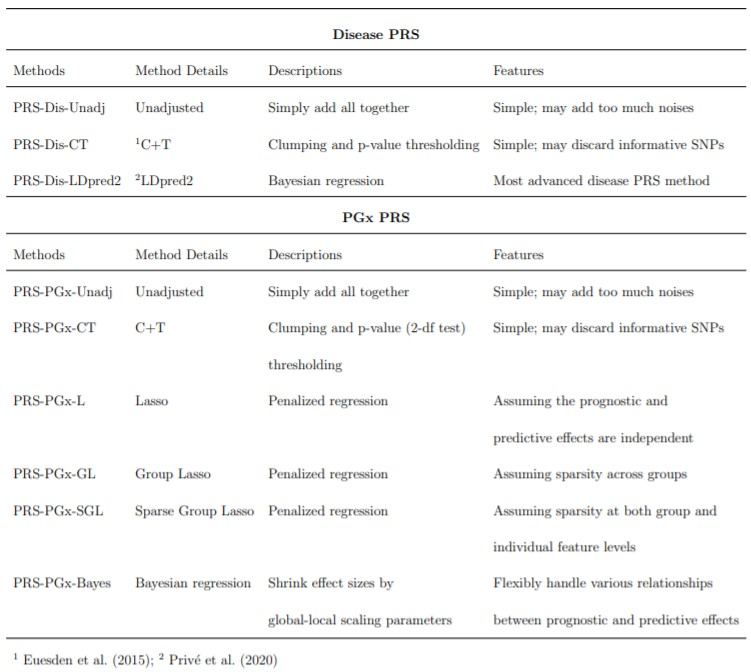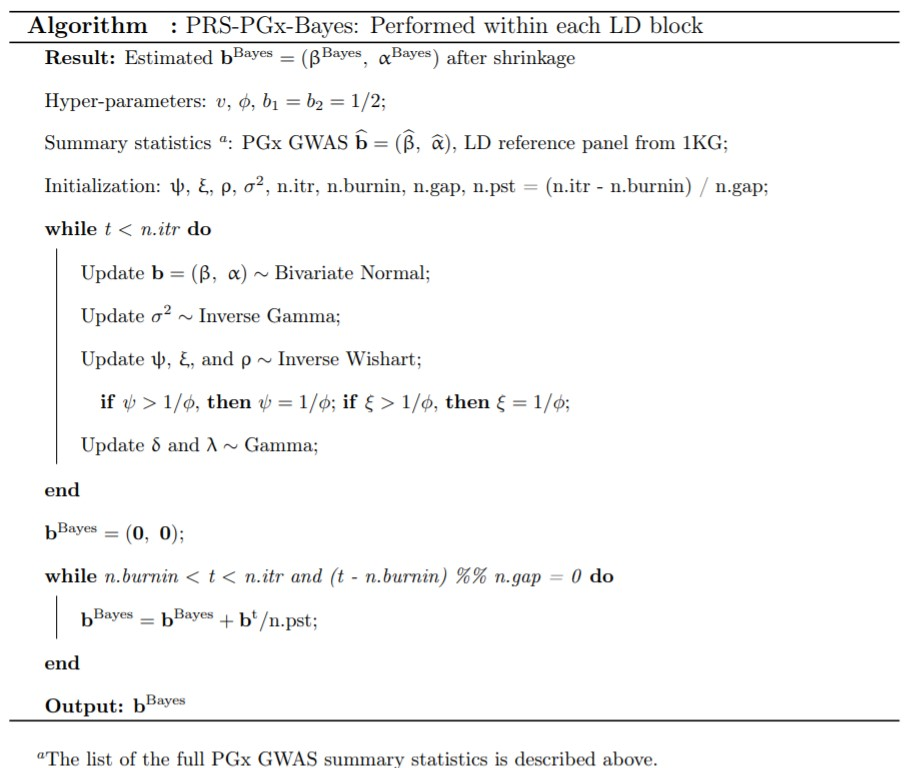# Pharmacogenomics Polygenic Risk Score for Drug Response Prediction Using PRS-PGx Methods

## Contents

• Overview
• System Requirements
• Installation Guide
• Demo
• References

## Overview

PRSPGx package (Zhai et al. 2021) implements a series of PRS (Polygenic Risk Score) methods for drug response prediction. They include novel PGx (pharmacogenomics) PRS (PRS-PGx) methods: PRS-PGx-CT (clumping and thresholding), PRS-PGx-Lasso (penalized regression), and PRS-PGx-Bayes (Bayesian regression) as well as the traditional disease PRS (PRS-DIS) methods: PRS-Dis-CT (clumping and thresholding), and PRS-Dis-LDpred2 (LDpred2).Table 1: Overview of PRS-DIS and PRS-PGx methods.

## System Requirements

The package development version is tested on the following systems:

Mac OSX: Mojave version 10.14.6 (R version 3.6.3)

Windows 10 (R version 3.6.3)

The CRAN package should be compatible with Windows and Mac operating systems.

## Installing Guide

PRSPGx package requires R with version 3.6.3 or higher, which can be downloaded and installed from CRAN.

### Package dependencies

Users should install the following packages prior to installing PRSPGx, from an R terminal:

install.packages(c('glmnet','gglasso','SGL','bigsnpr','bigstatsr','bigsparser',
'bigparallelr','MCMCpack','Matrix','GIGrvg','bdsmatrix',
'mvtnorm','lmtest','propagate','methods','Rfast','matrixcalc'))

The PRSPGx package functions with all packages in their latest versions as they appear on CRAN on July 18, 2022. The versions of software are, specifically:

gglasso (>= 1.5.0)
SGL (>= 1.3.0)
glmnet (>= 4.0.2)
bigsnpr (>= 1.5.2)
bigstatsr (>= 1.2.3)
Matrix (>= 1.2.18)
GIGrvg (>= 0.5.0)
MCMCpack (>= 1.4.6)
bdsmatrix (>= 1.3.4)
bigsparser (>= 0.4.0)
lmtest (>= 0.9.37)
mvtnorm (>= 1.1.0)
propagate (>= 1.0.6)
bigparallelr (>= 0.2.3)
methods (>= 3.6.3)
Rfast (>= 1.9.9)
matrixcalc (>= 1.0-3)

### Package Installation

After downloading PRSPGx package, unzip it and you will see PRSPGx_0.3.0.tar.gz. To install PRSPGx, type the following code from an R session:

install.package("PRSPGx_0.3.0.tar.gz", repos = NULL)
library(PRSPGx)

The package should take approximately 5 seconds to install.

## Demo

To apply our proposed PRS-DIS and PRS-PGx methods to the whole genome. It is recommended to run functions block by block (for example, LD blocks indicated by Berisa and Pickrell (2016)). In the following example, we consider a pseudo LD block with 1500 SNPs.

### Step 1: Prepare Summary Statistics and Reference Panel for Disease PRS and PGx PRS

In this section, we will load the simulated example data PRSPGx.example, in which the list includes the following elements:

• the disease GWAS summary statistics for PRS-DIS methods (DIS_GWAS), including SNP ID, position, minor allele frequency (MAF); p-value, marginal prognostic effect size estimate $$\widehat \beta$$, SE($$\widehat \beta$$), N;

• the PGx GWAS summary statistics for PRS-PGx methods (PGx_GWAS), including SNP ID, position, minor allele frequency (MAF); 2df (G + G $$\times$$ T) test p-value, marginal prognostic and predictive effect sizes estimates $$\widehat\beta$$ and $$\widehat \alpha$$, N; sd(Y) and mean(T);

• the simulated individual-level genotype as the reference panel matched with the PGx GWAS summary statistics (G_reference);

• the simulated phenotype (Y), treatment assignment (T), PGx sample genotype data (G), prognostic effect sizes (beta), and predictive effect sizes (alpha).

## Simulated sample example
data(PRSPGx.example); attach(PRSPGx.example)
## Training
## Individual-level data, prepared only for PRS-PGx-Lasso
Y_train <- Y[1:3000]; T_train <- Tr[1:3000]; G_train <- G[1:3000,]

## Testing
## Individual-level data
Y_test <- Y[3001:4000]; T_test <- Tr[3001:4000]; G_test <- G[3001:4000,]
## Performance Evaluation
run_eval <- function(coef_est, Y_test, T_test, G_test){
## Prognostic score
prog_score = as.vector(as.matrix(G_test)%*%coef_est$coef.G) ## Predictive score pred_score = as.vector(as.matrix(G_test)%*%coef_est$coef.TG)

## Performance evaluation
fit <- summary(lm(Y_test ~ T_test + prog_score + T_test:pred_score))
## prediction accuracy: r2
r2 = fit$adj.r.squared ## p-value of the interaction effect inter_pvalue = fit$coefficients[4,4]

result <- c(r2=r2, inter_pvalue=inter_pvalue)
return(result)
}

### Step 2. Run PRS-DIS Methods

#### 1. PRS-Dis-CT

The PRS-Dis-CT method constructs the prognostic PRS using the variant LD-clumping and p-value thresholding steps following Euesden, Lewis, and O’Reilly (2015). Specifically, for any pair of SNPs that have a physical distance smaller than 250 kb (default) and an $$r^2$$ greater than 0.8 (default), the less significant SNP is removed. The prognostic polygenic score is then calculated similarly to unadjusted approach. Disease PRS sets the predictive polygenic score directly equivalent to the prognostic score.

$\text{prognostic score = predictive score = } \sum_{j\in J} \mathbf G_{j}\hat{\beta}_j,$ where $$J$$ denotes the set of SNPs whose p-values from disease GWAS passing the threshold.

coef_est <- PRS_Dis_CT(DIS_GWAS, G_reference, pcutoff = 0.1, clumping = TRUE)
## Performance Evaluation
run_eval(coef_est, Y_test, T_test, G_test)
##           r2 inter_pvalue
##    0.2610913    0.9008115

#### 2. PRS-Dis-LDpred2

coef_est <- PRS_Dis_LDpred2(DIS_GWAS, G_reference, pcausal = 0.1, h2 = 0.4)
## Performance Evaluation
run_eval(coef_est, Y_test, T_test, G_test)
##           r2 inter_pvalue
##   0.26445393   0.03372538

### Step 3. Run PRS-PGx Methods

#### 1. PRS-PGx-CT

The PRS-PGx-CT method constructs the prognostic and predictive PRS simultaneously using the variant LD-clumping and 2-df p-value thresholding steps, similar to PRS-Dis-CT.

$\text{prognostic score = } \sum_{j\in J} \mathbf G_{j}\hat{\beta}_j,\quad \text{predictive score = } \sum_{j\in J} \mathbf G_{j}\hat{\alpha}_j,$ where $$J$$ denotes the set of SNPs whose 2-df p-values from PGx GWAS passing the threshold.

coef_est <- PRS_PGx_CT(PGx_GWAS, G_reference, pcutoff = 0.01, clumping = TRUE)
## Performance Evaluation
run_eval(coef_est, Y_test, T_test, G_test)
##           r2 inter_pvalue
## 3.155875e-01 1.702877e-18

#### 2. PRS-PGx-L, -GL, -SGL

Assume independence between prognostic and predictive effect sizes within each SNP, a direct solution is to minimize the following equation in the framework of Lasso (PRS-PGx-L):

$f(b)=\frac{1}{2} || \mathbf Y-\sum_{j=1}^m \mathbf X_j \mathbf b_j ||_2^2 + \lambda || \mathbf b ||_1,$ where $$\mathbf X_j=[\mathbf G_j,\ \mathbf{T\times G}_j]$$, and $$\mathbf b_j=(\beta_j,\ \alpha_j)$$. $$|| \cdot ||_1$$ and $$|| \cdot ||_2$$ stand for L1-norm and L2-norm, respectively. Assume if a SNP is included into the model, then both prognostic and predictive effects of that SNP will be non-zero, then Group Lasso (PRS-PGx-GL) might be appealing by considering each genetic marker as a group (Yang and Zou 2015):

$f(b)=\frac{1}{2} || \mathbf Y-\sum_{j=1}^m \mathbf X_j \mathbf b_j ||_2^2 + \lambda \sum_{j=1}^m \sqrt{p_j} || \mathbf b_j ||_2,$ where $$p_j=2$$ denotes the group size. Finally, if we assume sparsity at both the group and individual feature levels, we also consider the Sparse Group Lasso (PRS-PGx-SGL) whose penalty is a linear combination of penalties from Lasso and Group Lasso (Simon et al. 2015):

$f(b)=\frac{1}{2} || \mathbf Y-\sum_{j=1}^m \mathbf X_j \mathbf b_j ||_2^2 + \alpha\lambda || \mathbf b ||_1 + (1-\alpha) \lambda \sum_{j=1}^m \sqrt{p_j} || \mathbf b_j ||_2.$

## PRS-PGx-L (method = 1)
coef_est <- PRS_PGx_Lasso(Y_train, T_train, G_train, lambda = 1.1, method = 1)
## Performance Evaluation
run_eval(coef_est, Y_test, T_test, G_test)
##           r2 inter_pvalue
## 3.094038e-01 1.886631e-16
## PRS-PGx-GL (method = 2)
coef_est <- PRS_PGx_Lasso(Y_train, T_train, G_train, lambda = 0.5, method = 2)
## Performance Evaluation
run_eval(coef_est, Y_test, T_test, G_test)
##           r2 inter_pvalue
## 3.116102e-01 2.853173e-15
## PRS-PGx-SGL (method = 3)
coef_est <- PRS_PGx_Lasso(Y_train, T_train, G_train, lambda = 0.02, method = 3, alpha = 0.5)
## Performance Evaluation
run_eval(coef_est, Y_test, T_test, G_test)
##           r2 inter_pvalue
## 3.041532e-01 7.238016e-15

#### 3. PRS-PGx-Bayes

Motivated by Ge et al. (2019), PRS-PGx-Bayes (Bayesian regression) assigns priors on prognostic and predictive effect sizes as follows:

$(\beta_j,\ \alpha_j) \sim \text{MVN} (0,\ \frac{\sigma^2}{n}\phi M_j),\quad M_j= \begin{bmatrix} \psi_j & \rho_j\sqrt{\psi_j\xi_j} \\ \rho_j\sqrt{\psi_j\xi_j} & \xi_j \end{bmatrix}, \quad M_j\sim g(\cdot\ ,\ \cdot),$ where $$\phi$$ is a global scaling parameter that is shared across all effect sizes; $$\psi_j$$ and $$\xi_j$$ are local, marker-specific scaling parameters; $$\rho_j$$ is the marker-specific correlation between the two effect sizes $$\beta_j$$ and $$\alpha_j$$. The detailed algorithm is shown in Table 2.Table 2: PRS-PGx-Bayes algorithm.

paras = c(3, 5)
coef_est <- PRS_PGx_Bayes(PGx_GWAS, G_reference, n.itr = 100, n.burnin = 50, n.gap = 5, paras = paras)
## Performance Evaluation
run_eval(coef_est, Y_test, T_test, G_test)
##           r2 inter_pvalue
## 3.009385e-01 1.906163e-13